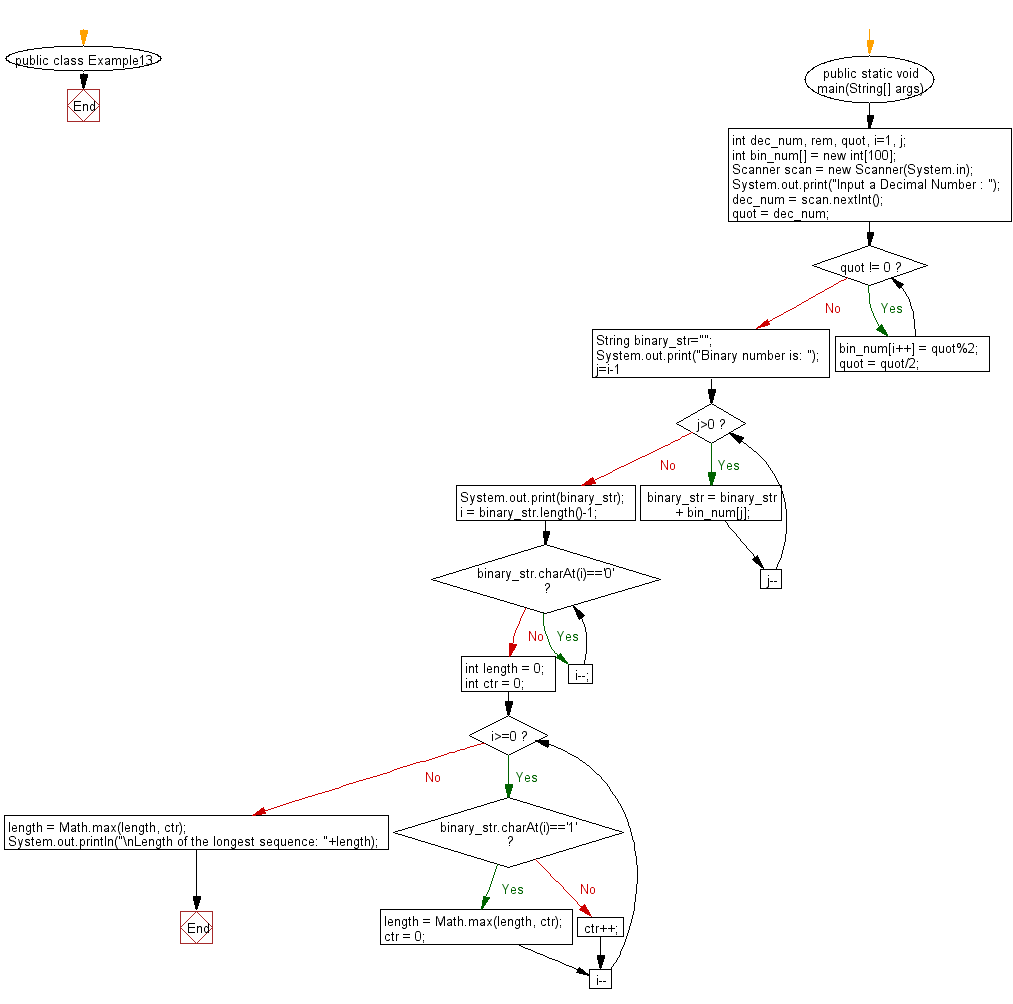﻿ Java Math Exercises: Find the length of the longest sequence of zeros in binary representation of an integer - w3resource# Java Math Exercises: Find the length of the longest sequence of zeros in binary representation of an integer

## Java Math Exercises: Exercise-13 with Solution

Write a Java program to find the length of the longest sequence of zeros in binary representation of an integer.

Sample example:
Number 7 has binary representation 111 and has no binary gaps.
Number 8 has binary representation 1000 and contains a binary gap of length 0.
Number 457 has binary representation 111001001 and contains a binary gap of length 2.
Number 40 has binary representation 101000 and contains one binary gap of length 1.
Number 12546 has binary representation 11000100000010 and contains highest binary gap of length 6.

Sample Solution:

Java Code:

``````import java.util.*;
public class Example13 {
public static void main(String[] args){

int dec_num, rem, quot, i=1, j;
int bin_num[] = new int;
Scanner scan = new Scanner(System.in);

System.out.print("Input a Decimal Number : ");
dec_num = scan.nextInt();

quot = dec_num;

while(quot != 0)
{
bin_num[i++] = quot%2;
quot = quot/2;
}
String binary_str="";
System.out.print("Binary number is: ");
for(j=i-1; j>0; j--)
{
binary_str = binary_str + bin_num[j];
}
System.out.print(binary_str);
i = binary_str.length()-1;
while(binary_str.charAt(i)=='0') {
i--;
}
int length = 0;
int ctr = 0;
for(; i>=0; i--) {
if(binary_str.charAt(i)=='1') {
length = Math.max(length, ctr);
ctr = 0;
} else {
ctr++;
}
}
length = Math.max(length, ctr);
System.out.println("\nLength of the longest sequence: "+length);
}
}
``````

Sample Output:

```Input a Decimal Number : 7
Binary number is: 111
Length of the longest sequence: 0
```

Flowchart:Java Code Editor:

What is the difficulty level of this exercise?

﻿

## Java: Tips of the Day

Array vs ArrayLists:

The main difference between these two is that an Array is of fixed size so once you have created an Array you cannot change it but the ArrayList is not of fixed size. You can create instances of ArrayLists without specifying its size. So if you create such instances of an ArrayList without specifying its size Java will create an instance of an ArrayList of default size.

Once an ArrayList is full it re-sizes itself. In fact, an ArrayList is internally supported by an array. So when an ArrayList is resized it will slow down its performance a bit as the contents of the old Array must be copied to a new Array.

At the same time, it's compulsory to specify the size of an Array directly or indirectly while creating it. And also Arrays can store both primitives and objects while ArrayLists only can store objects.

Ref: https://bit.ly/3o8L2KH